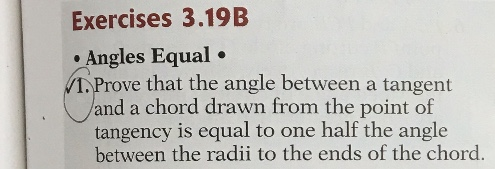# Exercises 3.19B• Angles Equal •1. Prove that the angle between a tangentland a chord drawn from the point oftangency is equal to one half the anglebetween the radii to the ends of the chord.

Question
4 views

Geometry Proof. Please write the answer like column proofs(statements and reasons), so I can understand it better.help_outlineImage TranscriptioncloseExercises 3.19B • Angles Equal • 1. Prove that the angle between a tangent land a chord drawn from the point of tangency is equal to one half the angle between the radii to the ends of the chord. fullscreen
check_circle

Step 1

Draw the diagram using the given information.

Step 2

To prove: Then angle BPQ is equal to one half of the angle PCQ.

Step 3

Consider the Triangle PCQ.

Since PC and CQ both are radius then PC = CQ.

So, Angle CPQ = Angle CQP = x bec...

### Want to see the full answer?

See Solution

#### Want to see this answer and more?

Solutions are written by subject experts who are available 24/7. Questions are typically answered within 1 hour.*

See Solution
*Response times may vary by subject and question.
Tagged in

### Circles